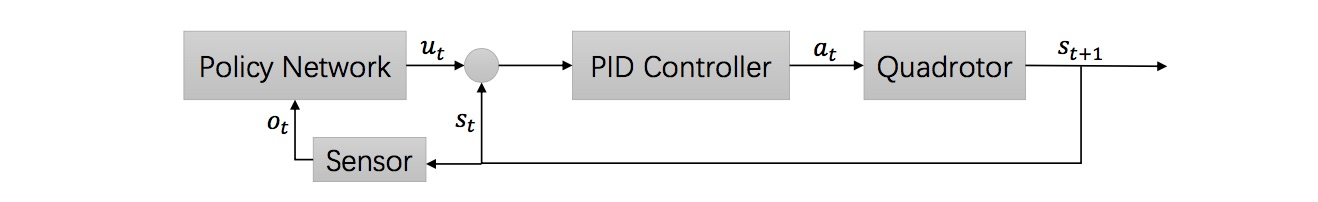# Training a Quadcopter to Autonomously Learn to Track

Posted by Chi Zhang on November 26, 2018

This a summary of our IJCAI 2018 paper in training a quadcopter to learn to track.

## 1. Introduction

We’ve witnessed the advent of a new era for robotics recently due to advances in control methods and reinforcement learning algorithms, where unmanned aerial vehicles (UAV) have demonstrated promising potential for both civil and commercial applications. Drones that fly meters above the ground provide an intermediate solution for navigation between car-based imaging and satellite-based imaging and assist in tracking fast-moving objects on the ground. These moving objects pose problems for other land vehicles because of obstacles, making a tracking system of drones desirable.

In this work, we consider the problem of learning to track for UAV. However, we take a different route from previous methods, where a perception module is used to estimate the target position and a rule-based controller is designed by human experts after tuning. Here, we adopt the recent achievement from deep reinforcement learning, combine it with control methods and train the entire system end-to-end.

## 2. Method Overview

In the work, a deep neural network is combined with a conventional proportional-integral-derivative (PID) controller in a hierarchical way such that the neural network provides the reference inputs for the PID control loop and the PID control module guides the rotor actions. We also propose to fuse the perception module and the control module together by a single neural network, which takes the raw images as input. For fast convergence, the visual processing component of the network is pretrained in a supervised manner first and fine-tuned later with the control layers by reinforcement learning. This training protocol realizes task performance optimization and the goal of target following.

## 3. The Approach

#### 3.1. Hierarchical Control of Neural Network and PID

Since the quadcopter features are highly non-linear and the aerodynamics of the drones are hard to learn directly from scratch, we propose to combine the neural network with the conventional PID control loop to tackle the problem. The following figure shows the high-level idea in designing the system.Figure 1. Hierarchical system combining the neural network with PID control.

At each time step $$t$$, given the observation $$o_t$$, the neural network produces a high-level feature vector $$u_t$$ for the PID controller, which further computes the corresponding low-level action signals $$a_t$$ for the rotors that guides the dynamics of the quadcopter.

#### 3.2. Supervised Learning for the Perception

While model-free reinforcement learning presents itself as a simple and direct approach to train a policy for complex tasks, it still suffers from low sample efficiency, meaning that training successful policies needs thousands, or even millions, of sample trajectories. This dramatically slows down the training phase.

To accelerate training, we assume supervised training that predicts mid-level targets could improve the efficiency. Concretely, we build a small dataset that pairs the image input with the mid-level target $$s_o$$ and train lower layers of our network in a supervised manner to predict these mid-level targets. The weights of the network are used for initialization and fine-tuned later for the reinforcement learning phase.

#### 3.3. Policy Gradient Reinforcement Learning

For the final reinforcement learning phase, we design an appropriate reward function and use the policy gradient method to train our network.

Formally, given the observation of the state $$o_t$$, the policy $$\pi_\theta(a_t \mid o_t)$$ parameterized by $$\theta$$ gives a distribution of the action (delta distribution if the policy is deterministic). An action is picked according to the distribution and executed in response to the observation. The quality of the action under the state is signaled by a reward function $$r_t(s_t, a_t)$$. Normally, reinforcement learning is also characterized by the transition distribution $$p(s_{t+1} \mid s_t, a_t)$$, but policy gradient methods relieve us from the pain of working out the transition dynamics. We define the return under the discounted scenario, $$R_t = \sum_{i=t}^T\gamma^{i - t}r(s_i, a_i)$$ where $$\gamma \in [0, 1]$$. The ultimate goal is to maximize the expected cumulative discounted reward $$\mathbb{E}_\pi[R_1]$$ following the policy $$\pi$$..

For the policy gradient, remember that our policy $$\pi$$ is parameterized by $$\theta$$ and thus a very straightforward way to optimize the expected total reward would be taking the gradient of the expectation with respect to the policy parameters and update the parameters in a gradient descent/ascent manner.

For a better introduction and survey of the policy gradient methods, please refer to  and .

## 4. Training Results

With all of our model components specified, we put all Lego pieces together and train our model end-to-end.

We first verify the superiority of our supervised pretraining phase by comparing it with other more standard neural network architectures and show its lower test error. We then visualize the learning process and test the model’s generalization ability. While we only train the model for up to 1,000 time steps for each episode during testing, the quadcopter closely tracks the target for more than 10,000 steps, i.e., 10 $$\times$$ more than the training phase. The model also performs relatively well under previously unseen scenes and when there are multiple similar targets acting as noise.

For experimental details, please refer to .

## 5. Discussion

Our experimental results have shown preliminary success of the algorithm. However, it still bears some limitations. A mature system should be tolerant to long-term occlusion and it’s desirable that a low-level mapping could be learned to replace the PID controller.